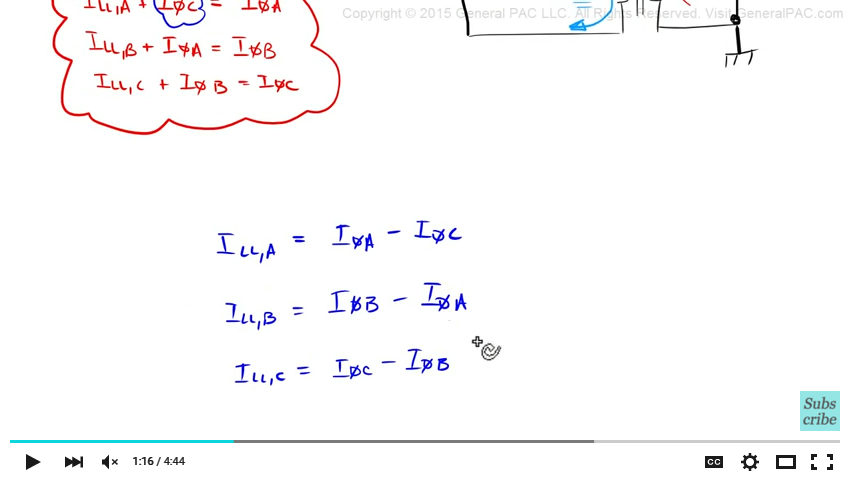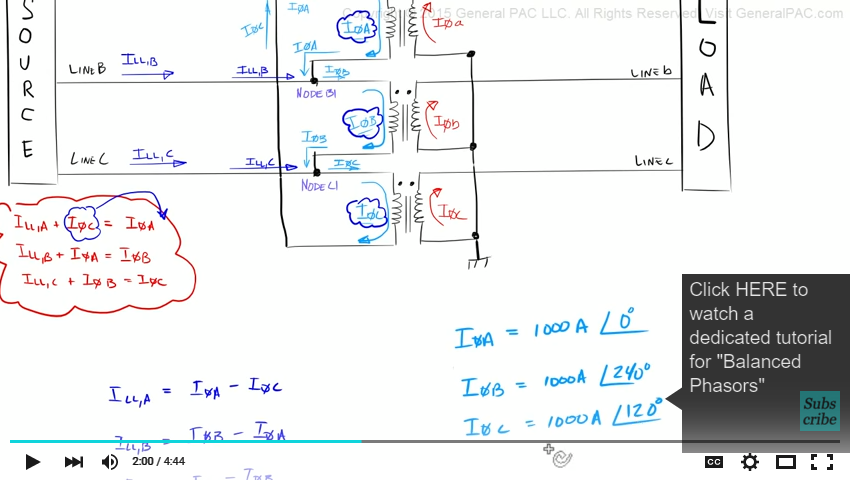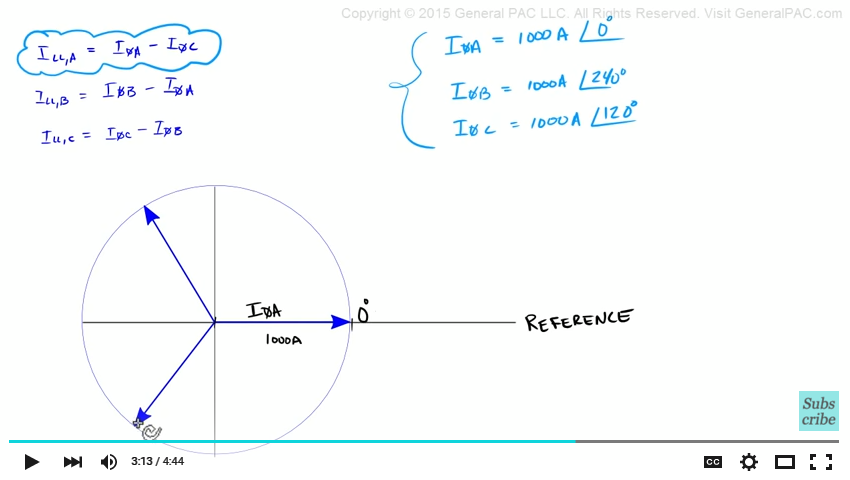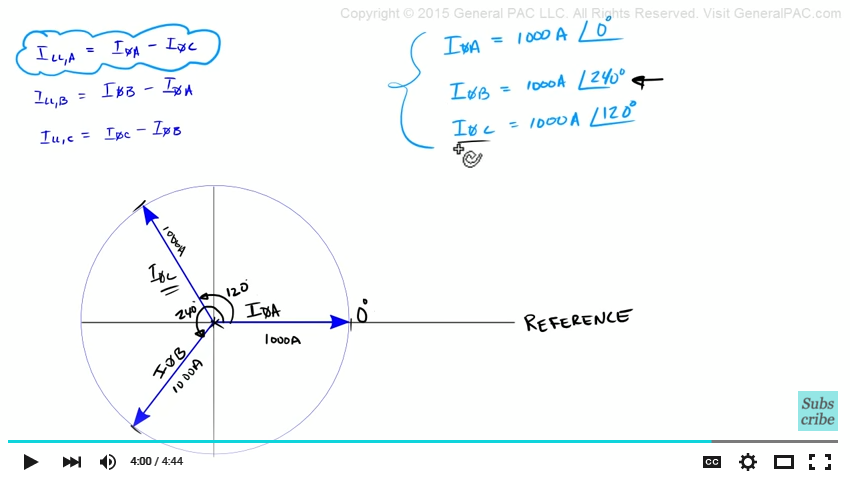This video was brought to you by GeneralPAC.com, making power systems Intuitive, Open and Free for Everyone, Everywhere. Consider subscribing and supporting through patreon.com/GeneralPAC. This is a mechanism for you to support us financially so we can continue making high quality power system video tutorials. Our corporate sponsor for this topic is AllumiaX.com from Seattle, Washington. Contact them for industrial and commercial power system studies.

Introduction to the Delta Wye Transformer connection. Part 5.

In part 5, we're going to perform phasor analysis on the Delta Wye transformer connection. And look at the square root of 3 and the 30 degrees phase shift come into play.

So this is what we illustrated in part 4. We did a nodal analysis on Node A1, Node B1, and Node C1. And looked at the relationship between line current and phase currents. In this equations, we said that the line current A plus the phase current C equals phase current A.

Now we're going to evaluate these particular equations and look at it from the phasor diagram's perspective. Let's rewrite these equations to something that make a little more sense. All we're going to do is move this phase current and move it to the other side of the equation. Okay so we're going to we-write this equation and solve for line current A.So line current A equals phase current A minus phase current C. Similarly, line current B equals phase current B minus phase current A. And Line current C equals phase current C minus phase current B.

Okay so now that we've written these equations like this, let's define our phase values. The phase values are the currents' that are flowing through our windings. We're going to define our phase A current, Phase B current, and phase C current.We're going to define these currents and create a balanced system. So let's say that phase current A on the primary side equals 100A at 0 degrees. And phase current B equals 1000A at 240 degrees. Phase current C equals 1000A at 120 degrees.

Ok so now we're going to draw these phasors and remember, these phasors are just the current that are flowing through the primary windings. Keep in mind the picture that we're trying to solve. We're performing phasor analysis because we want to relate this line current here with this phase current and this phase current. So we're doing a nodal analysis on Node A1. And what we're actually trying to solve with our phasor diagram is this particular equation. We're going to find out what this line current equal when we subtract phase current A minus phase current C.Okay this is what phasor diagram looks like. This line here at the 0 degree mark, this is our reference line. And this phasor that's going through our reference line which is at 0 degrees, this is phase A current. And what we're saying is that this origin point here to this point here, is 1000A. And the angle is at 0 degrees which is the same direction as our reference line.

This phasor here, this our phase B current. And from this origin here to this mark here is 1000A. And the angle, is what we defined here – 240 degrees. That angle is from this reference line going in the CCW direction, until we've gone 240 degrees. So this angle is 240 degrees. So we're defining this phasor B current here at the angle of 240 degrees.

And lastly, this phasor here is our phase C current. Again, from the origin to the end of the tip here is 1000A. And the angle from the reference line going CCW 120 degrees to get this point here.  So phase C current, which is this current here, equals 1000A at 120 degrees.So let's mark this angles here. We're saying this angle here, is 120 degrees. And this angle here is 240 degrees.

Okay we're going to take these phasors, and subtract phase A current with Phase C current and look at what our line current A looks like.

This video was brought to you by GeneralPAC.com. Making Power System Protection, Automation, and Controls intuitive.

#### Greetings from the GeneralPAC Team!

We make high-quality Power Systems Video Tutorials on complex topics that are free and open to everyone!  Thank you so much for supporting us through Patreon so we can continue doing good and valuable work.

What is Patreon and why do we use it?

Patreon is a fantastic portal that allows our fans and community to make monthly contribution (like Netflix subscription) so we can continue creating high-quality power systems video tutorials. In return, you get access to incredible perks like voting on future topics, getting your questions answered, access to VIP Q/A webinars with the creators of GeneralPAC, and much more! We THANK YOU for supporting us

Why do we need your support?

An incredible amount of time and effort is needed to develop high-quality video tutorials. Each video (Part 1 for example) takes approximately 10 hours to complete which includes learning the concept ourselves, brainstorming creative ways to teach and explain the concepts, writing the script, audio recording, video recording, and editing. It's no wonder why Hundreds-of-Thousands of people have watched, liked, subscribed, and left positive comments on Youtube channel. Your support truly makes all the difference.